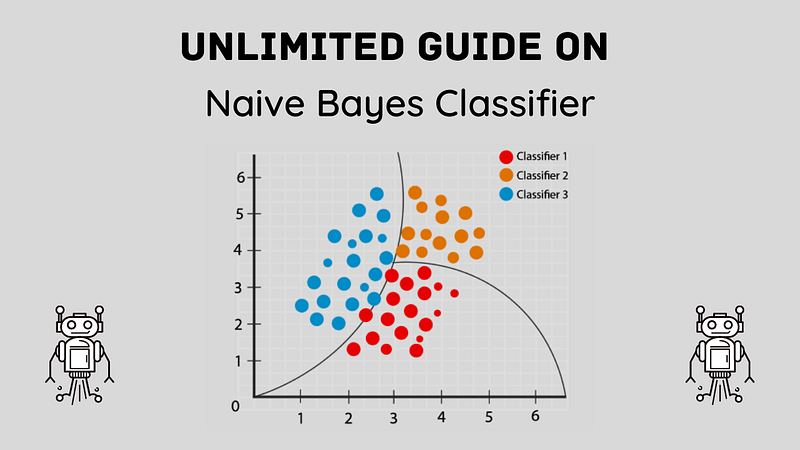# Naive Bayes Unleashed: A Comprehensive Guide to Probabilistic Machine Learning### Introduction

Machine Learning is the linchpin of modern Artificial Intelligence applications. It brings forth a myriad of algorithms designed to tackle various tasks ranging from simple regressions to complex deep learning. Among these algorithms, one has maintained its significance due to its simplicity, effectiveness, and versatility — Naive Bayes. The purpose of this detailed guide is to thoroughly explain the Naive Bayes algorithm, its working principles, its types, and its applications across various sectors.

### Understanding Naive Bayes

Naive Bayes is a popular classification technique based on Bayes’ Theorem with an assumption of independence among predictors. In simple terms, a Naive Bayes classifier assumes that the presence or absence of a particular feature of a class is unrelated to the presence or absence of any other feature. Despite its somewhat simplified assumptions, Naive Bayes classifiers often outperform more sophisticated classification methods.

### Bayes’ Theorem: The Foundation of Naive Bayes

The Naive Bayes algorithm is rooted in Bayes’ theorem, a fundamental theorem in the field of probability theory and statistics that describes the probability of an event based on prior knowledge of conditions related to the event. In mathematical terms:

`P(A|B) = P(B|A) * P(A) / P(B)`

Here, P(A|B) is the posterior probability of class (A, target) given predictor (B, attributes). P(A) is the prior probability of class. P(B|A) is the likelihood, which is the probability of the predictor given class. Lastly, P(B) is the prior probability of the predictor.

### How Does Naive Bayes Work?

The basic working mechanism of Naive Bayes revolves around the principle of maximum likelihood. It calculates the probability of an event in the following steps:

Step 1: Create a Frequency Table: The algorithm starts by creating a frequency table for each attribute against the target.

Step 2: Create a Likelihood Table: Next, it builds a likelihood table by finding the probabilities of given features for each class.

Step 3: Use Naive Bayesian Equation to Calculate Posterior Probability: For each class of the target variable, the algorithm calculates the posterior probability using Bayes theorem and naive assumption.

Step 4: Assign Class with the Highest Posterior Probability: The model predicts the class with the highest posterior probability as the outcome.

### Types of Naive Bayes Models

There are mainly three types of Naive Bayes models:

Gaussian: This model assumes that features follow a normal distribution. It’s used when the predictors take up a continuous value and are not discrete.

Multinomial: The multinomial Naive Bayes model is used for discrete counts. It’s often used in text classification problems where the data are typically represented as word vector counts.

Bernoulli: The Bernoulli Naive Bayes model is useful if your feature vectors are binary. It is also used for text classification tasks, but it considers whether or not a feature occurs.

### Applications of Naive Bayes Algorithms

Due to its simplicity and efficiency, Naive Bayes has found numerous applications in various sectors:

Text Classification/Spam Filtering/Sentiment Analysis: Naive Bayes classifiers are a popular choice for text classification tasks, which includes marking emails as spam or not, sentiment analysis, etc.

Recommendation System: Naive Bayes is used in recommendation systems that use machine learning and predictive modeling to offer suggestions to users based on their past behavior.

Medical and Health Care: It is used to predict different diseases based on the symptoms exhibited by patients.

Just like any other algorithm, Naive Bayes also has its strengths and weaknesses.

1. It’s easy and fast to predict the class of the test dataset. It also performs well in multi-class prediction.
2. When the assumption of independence holds, a Naive Bayes classifier performs better compared to other models like logistic regression.
3. It performs well in the case of categorical input variables compared to numerical variables.

1. The assumption of independent predictors: In real life, it’s almost impossible that we get a set of predictors that are completely independent.
2. If the categorical variable has a category in the test data set, which was not observed in the training data set, then the model will assign a 0 (zero) probability. This is often known as “Zero Frequency,” and to solve this, we can use the smoothing technique.

### Conclusion

Naive Bayes, though simplistic in its assumptions, remains one of the powerful machine learning algorithms, especially in tasks requiring probabilistic models. While it has some disadvantages, proper understanding and handling of the data, feature selection, and model tuning can mitigate these drawbacks to a great extent. This probabilistic algorithm, based on Bayes theorem, continues to hold its ground in the realm of machine learning and finds its utility in various applications.

# Personal Career & Learning Guide for Data Analyst, Data Engineer and Data Scientist

## Applied Machine Learning & Data Science Projects and Coding Recipes for Beginners

A list of FREE programming examples together with eTutorials & eBooks @ SETScholars

# Projects and Coding Recipes, eTutorials and eBooks: The best All-in-One resources for Data Analyst, Data Scientist, Machine Learning Engineer and Software Developer

Topics included:Classification, Clustering, Regression, Forecasting, Algorithms, Data Structures, Data Analytics & Data Science, Deep Learning, Machine Learning, Programming Languages and Software Tools & Packages.
(Discount is valid for limited time only)## Find more … …

Java tutorials for Beginners – Java Recursion

How to classify “wine” using SKLEARN Naïve Bayes models – Multiclass Classification in Python

Python Data Structure and Algorithm Tutorial – Master Theorem